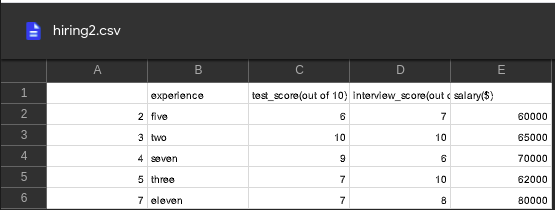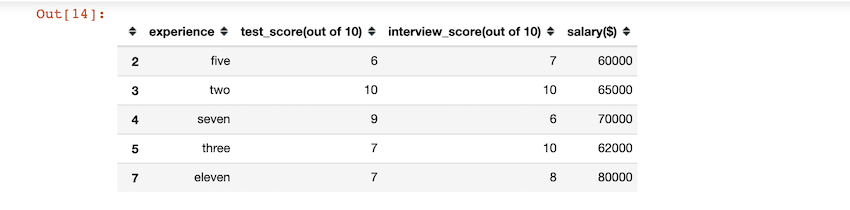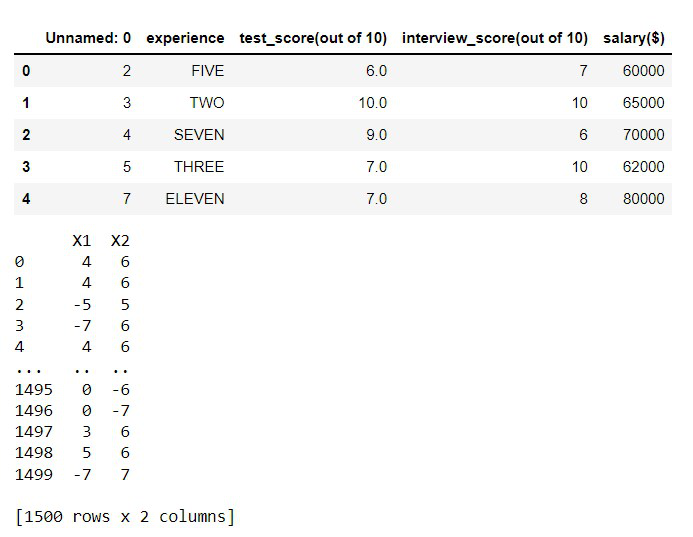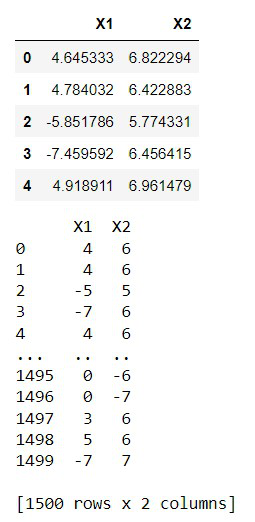# How to Use Pandas apply() inplace?

• Last Updated : 28 Nov, 2021

In this article, we are going to see how to use Pandas apply() inplace in Python.

In Python, this function is equivalent to the map() function. It takes a function as an input and applies it to a DataFrame as a whole. If you’re dealing with data in the form of tables, you’ll need to choose which axis your function should act on ( 0 for columns; and 1 for rows).

## Does the pandas apply() method have an inplace parameter?

No, the apply() method doesn’t contain an inplace parameter, unlike these pandas methods which have an inplace parameter:

• df.drop()
• df.rename( inplace=True)
• fillna()
• dropna()
• sort_values()
• reset_index()
• sort_index()
• rename()

## What actually does the inplace parameter mean?

The data is edited in place when inplace = True, which means it will return nothing and the data frame will be updated. When inplace = False, which is the default, the operation is carried out and a copy of the object is returned.

### Example 1: apply() inplace for One Column

in the below code. we first imported the pandas package and imported our CSV file using pd.read_csv(). after importing we use the apply function on the ‘experience’ column of our data frame. we convert the strings of that column to uppercase.

Used CSV file:## Python3

 `# code ` `import` `pandas as pd ` ` `  `# importing our dataset ` `df ``=` `pd.read_csv(``'hiring.csv'``) ` `# viewing the dataFrame ` `print``(df) ` ` `  `# we change the case of all the strings ` `# in experience column to uppercase ` `df[``'experience'``] ``=` `df[``'experience'``].``apply``(``str``.upper) ` ` `  `# viewing the modified column ` `print``(df[``'experience'``]) `

Output:```0      FIVE
1       TWO
2     SEVEN
3     THREE
4    ELEVEN
Name: experience, dtype: object```

### Example 2: apply() inplace for Multiple Columns

In this example, we use apply() method on multiple columns. we change the datatype of the columns from float to int. Used CSV file click here.

## Python3

 `import` `pandas as pd ` `import` `numpy as np ` ` `  `# importing our dataset ` `data ``=` `pd.read_csv(``'cluster_blobs.csv'``) ` ` `  `# viewing the dataFrame ` `print``(df) ` ` `  `# we convert the datatype of columns from float to int. ` `data[[``'X1'``, ``'X2'``]] ``=` `data[[``'X1'``, ``'X2'``]].``apply``(np.int64) ` ` `  `# viewing the modified column ` `print``(data[[``'X1'``, ``'X2'``]]) `

Output:### Example 3: apply() inplace for All Columns.

in this example, we use the same CSV file as before. here we use the apply() method on the entire data frame. we change the datatype of the columns from float to int.

## Python3

 `import` `pandas as pd ` `import` `numpy as np ` ` `  `# importing our dataset ` `data ``=` `pd.read_csv(``'cluster_blobs.csv'``) ` ` `  `# viewing the dataFrame ` `print``(data) ` ` `  `# we convert the datatype of ` `# columns from float to int. ` `data ``=` `data.``apply``(np.int64) ` ` `  `# viewing the modified column ` `print``(data) `

Output:My Personal Notes arrow_drop_up
Recommended Articles
Page :